# 8.7 Parametric equations: graphs  (Page 3/4)

 Page 3 / 4

Access the following online resource for additional instruction and practice with graphs of parametric equations.

## Key concepts

• When there is a third variable, a third parameter on which $\text{\hspace{0.17em}}x\text{\hspace{0.17em}}$ and $\text{\hspace{0.17em}}y\text{\hspace{0.17em}}$ depend, parametric equations can be used.
• To graph parametric equations by plotting points, make a table with three columns labeled $\text{\hspace{0.17em}}t,x\left(t\right),\text{\hspace{0.17em}}$ and $\text{\hspace{0.17em}}y\left(t\right).\text{\hspace{0.17em}}$ Choose values for $\text{\hspace{0.17em}}t\text{\hspace{0.17em}}$ in increasing order. Plot the last two columns for $\text{\hspace{0.17em}}x\text{\hspace{0.17em}}$ and $\text{\hspace{0.17em}}y.\text{\hspace{0.17em}}$ See [link] and [link] .
• When graphing a parametric curve by plotting points, note the associated t -values and show arrows on the graph indicating the orientation of the curve. See [link] and [link] .
• Parametric equations allow the direction or the orientation of the curve to be shown on the graph. Equations that are not functions can be graphed and used in many applications involving motion. See [link] .
• Projectile motion depends on two parametric equations: $\text{\hspace{0.17em}}x=\left({v}_{0}\mathrm{cos}\text{\hspace{0.17em}}\theta \right)t\text{\hspace{0.17em}}$ and $\text{\hspace{0.17em}}y=-16{t}^{2}+\left({v}_{0}\mathrm{sin}\text{\hspace{0.17em}}\theta \right)t+h.\text{\hspace{0.17em}}$ Initial velocity is symbolized as $\text{\hspace{0.17em}}{v}_{0}.\text{\hspace{0.17em}}\theta$ represents the initial angle of the object when thrown, and $\text{\hspace{0.17em}}h\text{\hspace{0.17em}}$ represents the height at which the object is propelled.

## Verbal

What are two methods used to graph parametric equations?

plotting points with the orientation arrow and a graphing calculator

What is one difference in point-plotting parametric equations compared to Cartesian equations?

Why are some graphs drawn with arrows?

The arrows show the orientation, the direction of motion according to increasing values of $\text{\hspace{0.17em}}t.$

Name a few common types of graphs of parametric equations.

Why are parametric graphs important in understanding projectile motion?

The parametric equations show the different vertical and horizontal motions over time.

## Graphical

For the following exercises, graph each set of parametric equations by making a table of values. Include the orientation on the graph.

$\left\{\begin{array}{l}x\left(t\right)=t\hfill \\ y\left(t\right)={t}^{2}-1\hfill \end{array}$

 $t$ $x$ $y$ $-3$ $-2$ $-1$ $0$ $1$ $2$ $3$

$\left\{\begin{array}{l}x\left(t\right)=t-1\hfill \\ y\left(t\right)={t}^{2}\hfill \end{array}$

 $t$ $-3$ $-2$ $-1$ $0$ $1$ $2$ $x$ $y$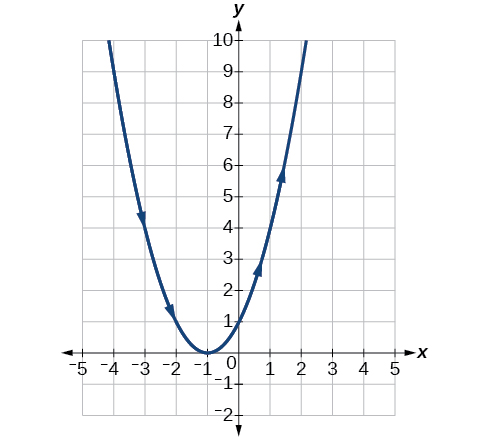$\left\{\begin{array}{l}x\left(t\right)=2+t\hfill \\ y\left(t\right)=3-2t\hfill \end{array}$

 $t$ $-2$ $-1$ $0$ $1$ $2$ $3$ $x$ $y$

$\left\{\begin{array}{l}x\left(t\right)=-2-2t\hfill \\ y\left(t\right)=3+t\hfill \end{array}$

 $t$ $-3$ $-2$ $-1$ $0$ $1$ $x$ $y$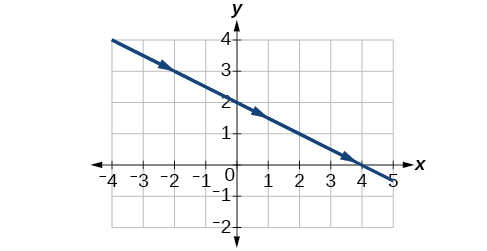$\left\{\begin{array}{l}x\left(t\right)={t}^{3}\hfill \\ y\left(t\right)=t+2\hfill \end{array}$

 $t$ $-2$ $-1$ $0$ $1$ $2$ $x$ $y$

$\left\{\begin{array}{l}x\left(t\right)={t}^{2}\hfill \\ y\left(t\right)=t+3\hfill \end{array}$

 $t$ $-2$ $-1$ $0$ $1$ $2$ $x$ $y$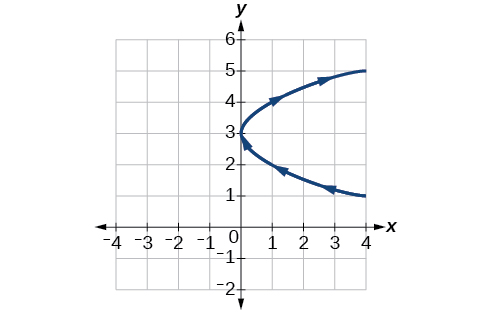For the following exercises, sketch the curve and include the orientation.

$\left\{\begin{array}{l}x\left(t\right)=t\\ y\left(t\right)=\sqrt{t}\end{array}$

$\left\{\begin{array}{l}x\left(t\right)=-\text{\hspace{0.17em}}\sqrt{t}\\ y\left(t\right)=t\end{array}$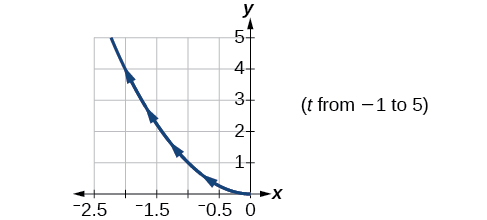$\left\{\begin{array}{l}x\left(t\right)=5-|t|\\ y\left(t\right)=t+2\end{array}$

$\left\{\begin{array}{l}x\left(t\right)=-t+2\\ y\left(t\right)=5-|t|\end{array}$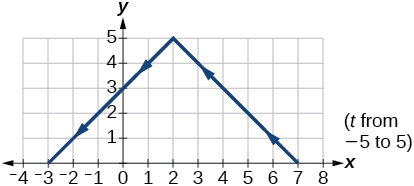$\left\{\begin{array}{l}x\left(t\right)=4\text{sin}\text{\hspace{0.17em}}t\hfill \\ y\left(t\right)=2\mathrm{cos}\text{\hspace{0.17em}}t\hfill \end{array}$

$\left\{\begin{array}{l}x\left(t\right)=2\text{sin}\text{\hspace{0.17em}}t\hfill \\ y\left(t\right)=4\text{cos}\text{\hspace{0.17em}}t\hfill \end{array}$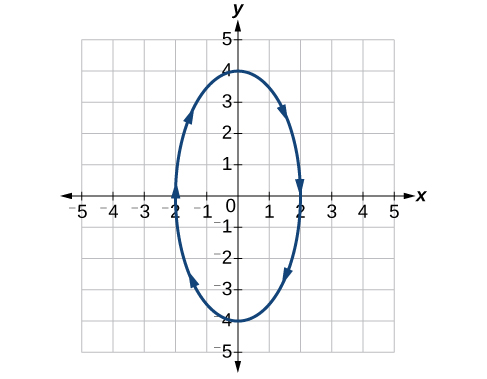$\left\{\begin{array}{l}x\left(t\right)=3{\mathrm{cos}}^{2}t\\ y\left(t\right)=-3\mathrm{sin}\text{\hspace{0.17em}}t\end{array}$

$\left\{\begin{array}{l}x\left(t\right)=3{\mathrm{cos}}^{2}t\\ y\left(t\right)=-3{\mathrm{sin}}^{2}t\end{array}$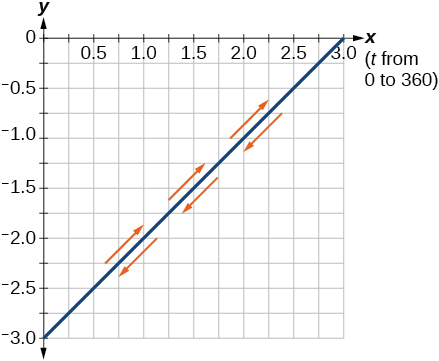$\left\{\begin{array}{l}x\left(t\right)=\mathrm{sec}\text{\hspace{0.17em}}t\\ y\left(t\right)=\mathrm{tan}\text{\hspace{0.17em}}t\end{array}$

$\left\{\begin{array}{l}x\left(t\right)=\mathrm{sec}\text{\hspace{0.17em}}t\\ y\left(t\right)={\mathrm{tan}}^{2}t\end{array}$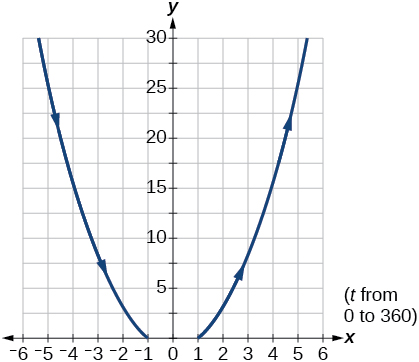$\left\{\begin{array}{l}x\left(t\right)=\frac{1}{{e}^{2t}}\\ y\left(t\right)={e}^{-\text{\hspace{0.17em}}t}\end{array}$

For the following exercises, graph the equation and include the orientation. Then, write the Cartesian equation.

$\left\{\begin{array}{l}x\left(t\right)=t-1\hfill \\ y\left(t\right)=-{t}^{2}\hfill \end{array}$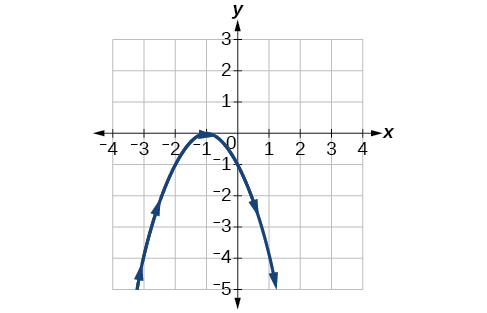$\left\{\begin{array}{l}x\left(t\right)={t}^{3}\hfill \\ y\left(t\right)=t+3\hfill \end{array}$

$\left\{\begin{array}{l}x\left(t\right)=2\mathrm{cos}\text{\hspace{0.17em}}t\\ y\left(t\right)=-\mathrm{sin}\text{\hspace{0.17em}}t\end{array}$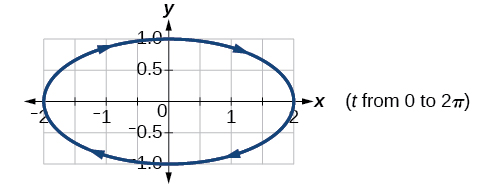$\left\{\begin{array}{l}x\left(t\right)=7\mathrm{cos}\text{\hspace{0.17em}}t\\ y\left(t\right)=7\mathrm{sin}\text{\hspace{0.17em}}t\end{array}$

$\left\{\begin{array}{l}x\left(t\right)={e}^{2t}\\ y\left(t\right)=-{e}^{\text{\hspace{0.17em}}t}\end{array}$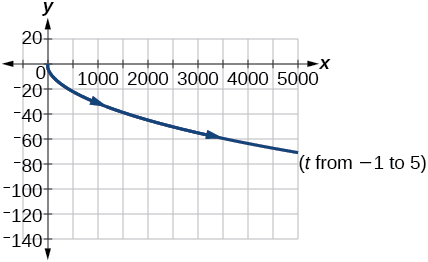For the following exercises, graph the equation and include the orientation.

$x={t}^{2},\text{\hspace{0.17em}}y\text{\hspace{0.17em}}=\text{\hspace{0.17em}}3t,\text{\hspace{0.17em}}0\le t\le 5$

$x=2t,\text{\hspace{0.17em}}y\text{\hspace{0.17em}}=\text{\hspace{0.17em}}\text{\hspace{0.17em}}{t}^{2},\text{\hspace{0.17em}}-5\le t\le 5$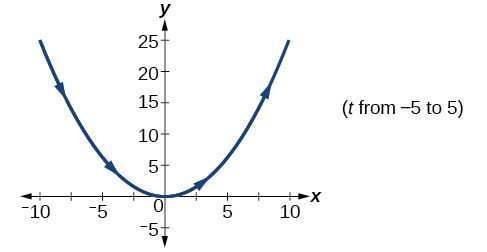$x=t,\text{\hspace{0.17em}}y=\sqrt{25-{t}^{2}},\text{\hspace{0.17em}}0

$x\left(t\right)=-t,y\left(t\right)=\sqrt{t},\text{\hspace{0.17em}}t\ge 0$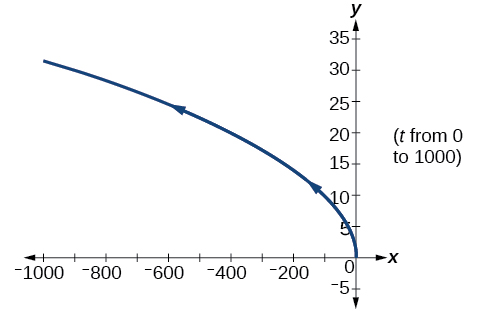$x=-2\mathrm{cos}\text{\hspace{0.17em}}t,\text{\hspace{0.17em}}y=6\text{\hspace{0.17em}}\mathrm{sin}\text{\hspace{0.17em}}t,\text{\hspace{0.17em}}0\le t\le \pi$

$x=-\mathrm{sec}\text{\hspace{0.17em}}t,\text{\hspace{0.17em}}y=\mathrm{tan}\text{\hspace{0.17em}}t,\text{\hspace{0.17em}}-\frac{\text{\hspace{0.17em}}\pi }{2}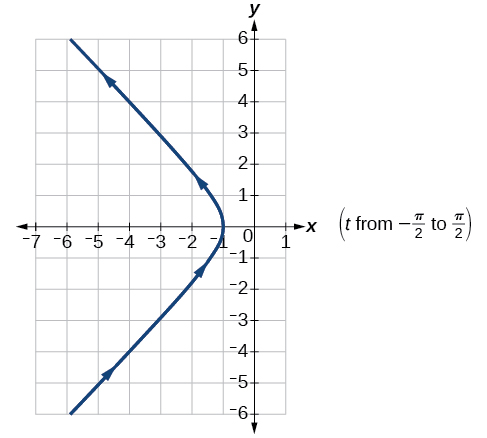For the following exercises, use the parametric equations for integers a and b :

$\begin{array}{l}x\left(t\right)=a\mathrm{cos}\left(\left(a+b\right)t\right)\\ y\left(t\right)=a\mathrm{cos}\left(\left(a-b\right)t\right)\end{array}$

Graph on the domain $\text{\hspace{0.17em}}\left[-\pi ,0\right],\text{\hspace{0.17em}}$ where $\text{\hspace{0.17em}}a=2\text{\hspace{0.17em}}$ and $\text{\hspace{0.17em}}b=1,\text{\hspace{0.17em}}$ and include the orientation.

Graph on the domain $\text{\hspace{0.17em}}\left[-\pi ,0\right],\text{\hspace{0.17em}}$ where $\text{\hspace{0.17em}}a=3\text{\hspace{0.17em}}$ and $\text{\hspace{0.17em}}b=2$ , and include the orientation.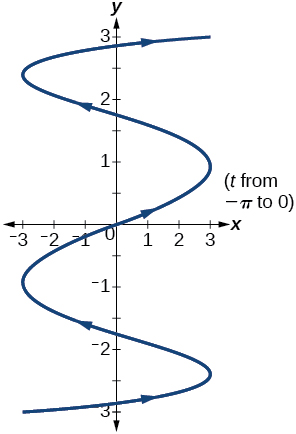Graph on the domain $\text{\hspace{0.17em}}\left[-\pi ,0\right],\text{\hspace{0.17em}}$ where $\text{\hspace{0.17em}}a=4\text{\hspace{0.17em}}$ and $\text{\hspace{0.17em}}b=3$ , and include the orientation.

a colony of bacteria is growing exponentially doubling in size every 100 minutes. how much minutes will it take for the colony of bacteria to triple in size
I got 300 minutes. is it right?
Patience
no. should be about 150 minutes.
Jason
what is the importance knowing the graph of circular functions?
can get some help basic precalculus
What do you need help with?
Andrew
how to convert general to standard form with not perfect trinomial
can get some help inverse function
ismail
Rectangle coordinate
how to find for x
it depends on the equation
Robert
whats a domain
The domain of a function is the set of all input on which the function is defined. For example all real numbers are the Domain of any Polynomial function.
Spiro
foci (–7,–17) and (–7,17), the absolute value of the differenceof the distances of any point from the foci is 24.
difference between calculus and pre calculus?
give me an example of a problem so that I can practice answering
x³+y³+z³=42
Robert
dont forget the cube in each variable ;)
Robert
of she solves that, well ... then she has a lot of computational force under her command ....
Walter
what is a function?
I want to learn about the law of exponent
explain this
what is functions?
A mathematical relation such that every input has only one out.
Spiro
yes..it is a relationo of orders pairs of sets one or more input that leads to a exactly one output.
Mubita
Is a rule that assigns to each element X in a set A exactly one element, called F(x), in a set B.
RichieRich
If the plane intersects the cone (either above or below) horizontally, what figure will be created?

#### Get Jobilize Job Search Mobile App in your pocket Now!ByByBy OpenStaxBy Robert MurphyBy Jazzycazz JacksonBy OpenStaxBy Jesenia WoffordBy Maureen MillerBy OpenStaxBy OpenStaxBy OpenStaxBy Janet Forrester